# How to Calculate and Solve for Pouring Speed | Design of Gating System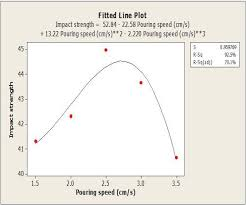The image above represents pouring speed.

To compute for poring speed, three essential parameters are needed and these parameters are Co-efficient for friction (μ), Acceleration due to Gravity (g) and Rated head static (Hs).

The formula for calculating pouring speed:

v = μ√(2gHs)

Where:

v = Pouring Speed
μ = Co-efficient for Friction
g = Acceleration due to Gravity

Let’s solve an example;
Find the pouring speed when the co-efficient for friction is 14, acceleration due to gravity is 9 and rated static head is 16.

This implies that;

μ = Co-efficient for Friction = 14
g = Acceleration due to Gravity = 9
Hs = Rated Static Head = 16

v = μ√(2gHs)
v = 14 x √(2 x 9 x 16)
v = 14 x √(288)
v = 14 x 16.97
v = 237.58

Therefore, the pouring speed is 237.58 m/s.

Calculating the Co-efficient for Friction when the Pouring Speed, the Acceleration due to Gravity and the Rated Static Head is Given.

μ = v / √(2gHs)

Where;

μ = Co-efficient for Friction
v = Pouring Speed
g = Acceleration due to Gravity

Let’s solve an example;
Find the co-efficient for friction when the pouring speed is 24, the acceleration due to gravity is 9 and the rated static head is 11.

This implies that;

v = Pouring Speed = 24
g = Acceleration due to Gravity = 9
Hs = Rated Static Head = 11

μ = v / √(2gHs)
μ = 24 / √(2 x 9 x 11)
μ = 24 / √198
μ = 24 / 14.07
μ = 1.70

Therefore, the co-efficient for friction is 1.70.

Calculating the Acceleration due to Gravity when the Pouring Speed, the Co-efficient for Friction and the Rated Static Head is Given.

g = (v / μ)2 / 2Hs

Where;

g = Acceleration due to Gravity
v = Pouring Speed
μ = Co-efficient for Friction

Let’s solve an example;
Find the acceleration due to gravity when the pouring speed is 22, the co-efficient for friction is 10 and the rated static head is 14.

This implies that;

v = Pouring Speed = 22
μ = Co-efficient for Friction = 10
Hs = Rated Static Head = 14

g = (v / μ)2 / 2Hs
g = (22 / 10)2 / 2 x 14
g = (2.2)2 / 28
g = 4.84 / 28
g = 0.172

Therefore, the acceleration due to gravity is 0.172.

Calculating the Rated Static Head when the Pouring Speed, the Co-efficient for Friction and the Acceleration due to Gravity is Given.

Hs = (v / μ)2 / 2g

Where;

v = Pouring Speed
μ = Co-efficient for Friction
g = Acceleration due to Gravity

Let’s solve an example;
Find the rated static head when the pouring speed is 30, the co-efficient for friction is 10 and the acceleration due to gravity is 8.

This implies that;

v = Pouring Speed = 30
μ = Co-efficient for Friction = 10
g = Acceleration due to Gravity = 8

Hs = (v / μ)2 / 2g
Hs = (30 / 10)2 / 2 x 8
Hs = (3)2 / 16
Hs = 9 / 16
Hs = 0.56

Therefore, the rated static head is 0.56.

Nickzom Calculator – The Calculator Encyclopedia is capable of calculating the pouring speed.

To get the answer and workings of the pouring speed using the Nickzom Calculator – The Calculator Encyclopedia. First, you need to obtain the app.

You can get this app via any of these means:

To get access to the professional version via web, you need to register and subscribe for NGN 1,500 per annum to have utter access to all functionalities.
You can also try the demo version via https://www.nickzom.org/calculator

Apple (Paid) – https://itunes.apple.com/us/app/nickzom-calculator/id1331162702?mt=8
Once, you have obtained the calculator encyclopedia app, proceed to the Calculator Map, then click on Materials & Metallurgical under Engineering.Now, Click on Foundry Technology under Material & MetallurgicalNow, Click on Design of Gating System under Foundry TechnologyNow, Click on Pouring Speed under Design of Gating System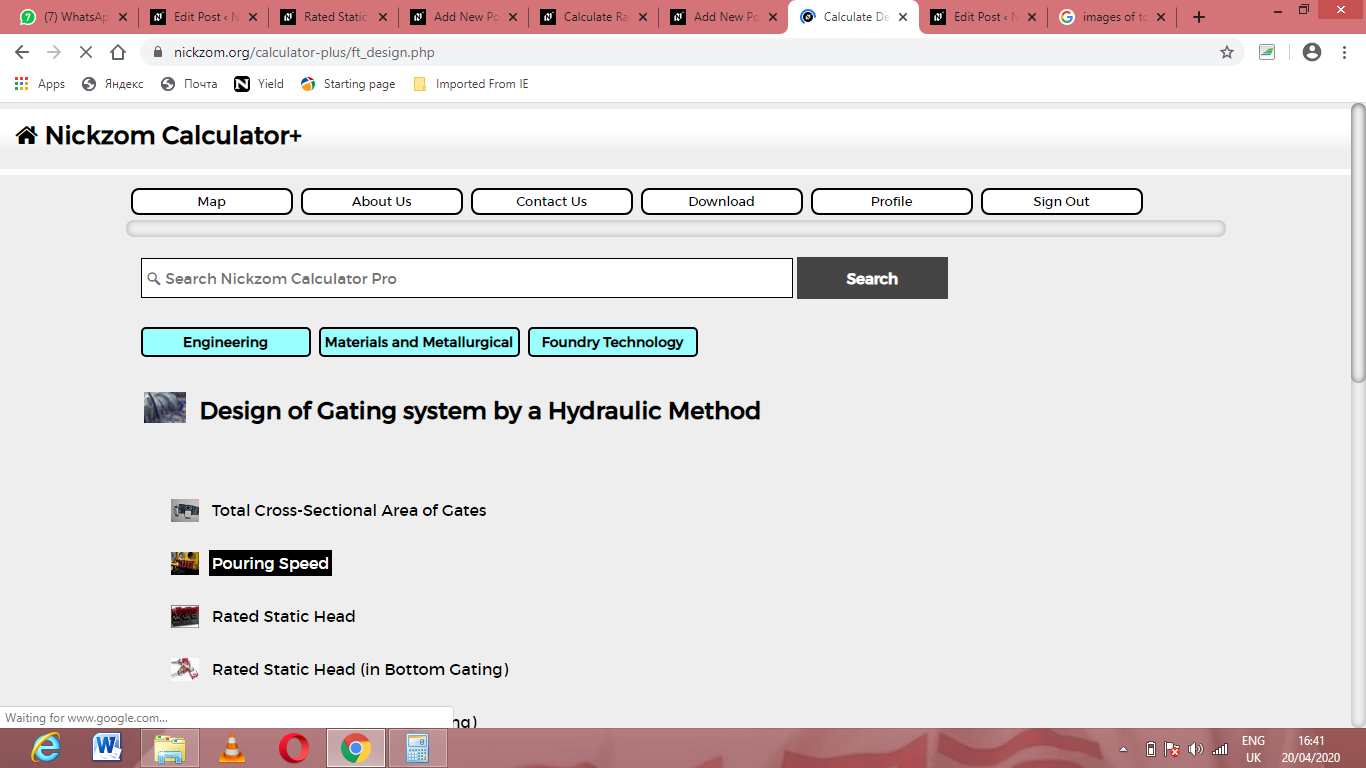The screenshot below displays the page or activity to enter your values, to get the answer for the pouring speed according to the respective parameters which are the Co-efficient for friction (μ), Acceleration due to Gravity (g) and Rated head static (Hs).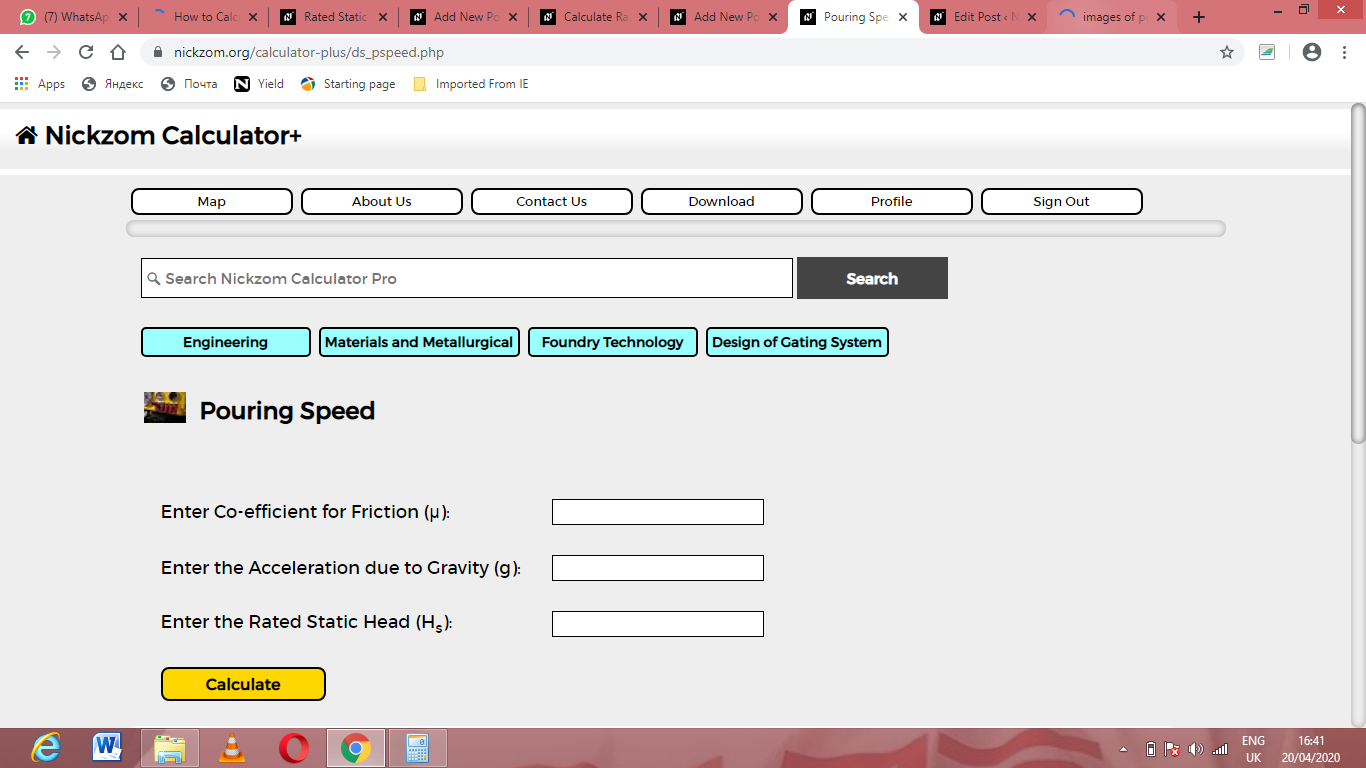Now, enter the values appropriately and accordingly for the parameters as required by the Co-efficient for friction (μ) is 14, Acceleration due to Gravity (g) is 9 and Rated head static (Hs) is 16.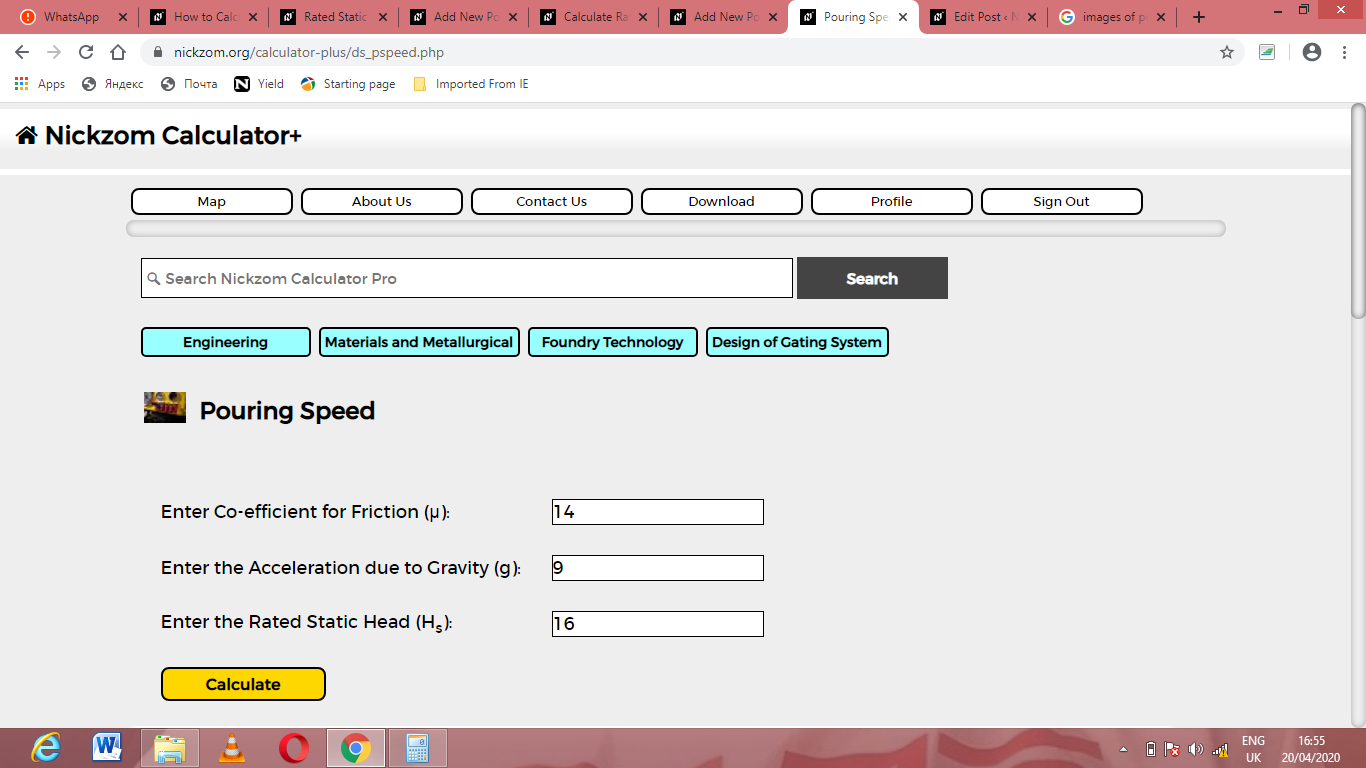Finally, Click on Calculate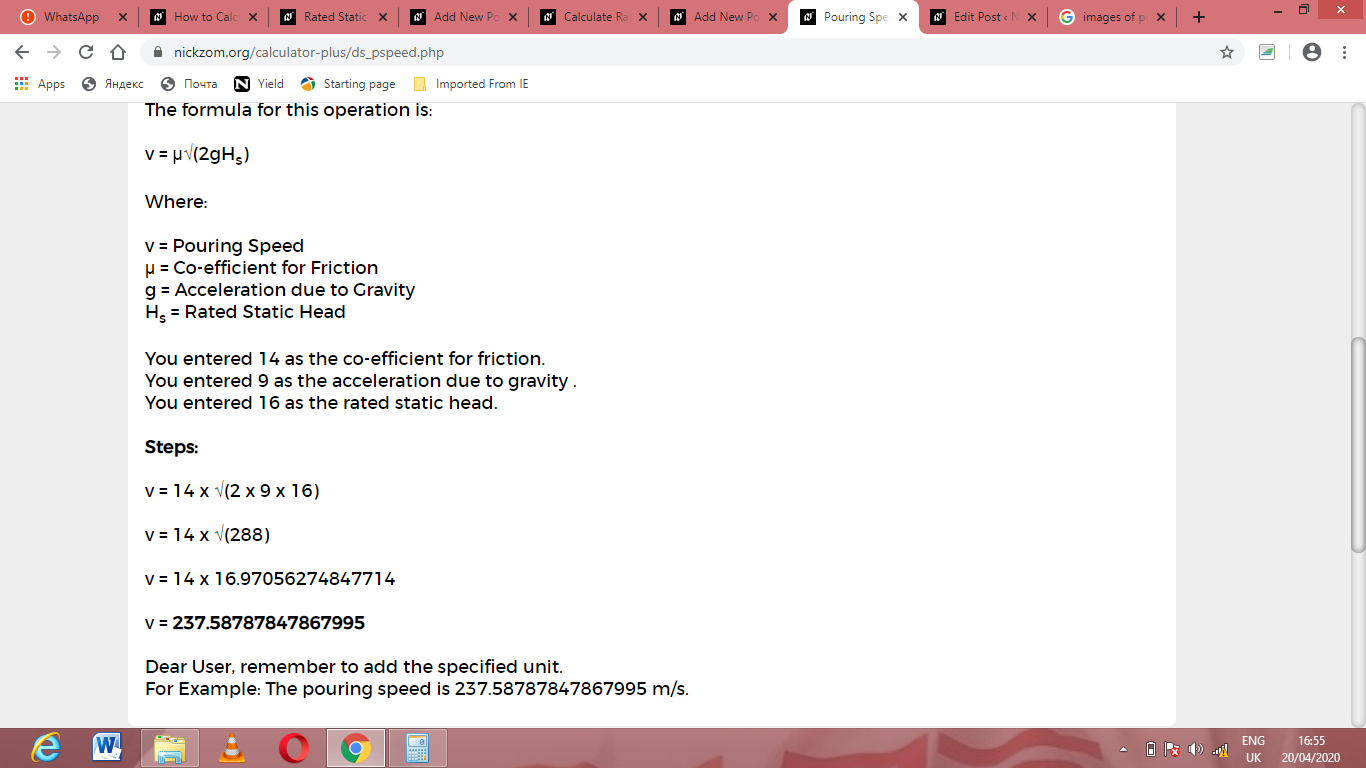As you can see from the screenshot above, Nickzom Calculator– The Calculator Encyclopedia solves for the pouring speed and presents the formula, workings and steps too.